# ISEE Upper Level Quantitative : Variables

## Example Questions

### Example Question #21 : Variables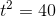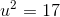Evaluate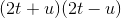.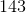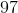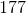Explanation:is the product of the sum and the difference of the same two binomials, so this can be rewritten, and evaluated, using the difference of squares pattern: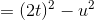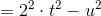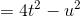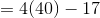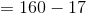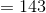### Example Question #1 : How To Divide Variables

The ratio of 10 to 14 is closest to what value?

0.71

0.57

0.04

0.24

0.71

Explanation:

Another way to express ratios is through division. 10 divided by 14 is approximate 0.71.

### Example Question #21 : Operations

If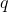is the quotient ofand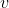, which statement could be true?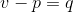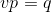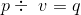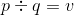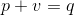Explanation:

A quotient is the result of division. Ifis the quotient ofand, that means that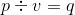could be true.

### Example Question #22 : Operationsis a negative integer. Which is the greater quantity?

(A)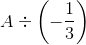(B)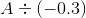(A) and (B) are equal

(A) is greater

(B) is greater

It is impossible to tell which is greater from the information given

(B) is greater

Explanation:

Since the quotient of negative numbers is positive, both results will be positive.

We can rewrite both of these as products of positive numbers, as follows: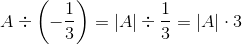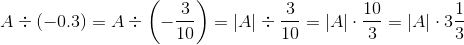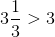, so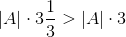, and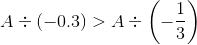making (B) greater.

### Example Question #4 : How To Divide Variablesis a negative integer. Which is the greater quantity?

(A)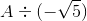(B)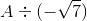It is impossible to determine which is greater from the information given

(A) is greater

(B) is greater

(A) and (B) are equal

(A) is greater

Explanation:

Since the quotient of negative numbers is positive, both results will be positive.

We can rewrite both of these as quotients of positive numbers, as follows: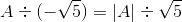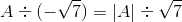Since the expressions have the same dividend and the second has the greater divisor, the first has the greater quotient.

Therefore, (A) is greater.

### Example Question #22 : Variables

Letbe negative. Which of the following is the greater quantity?

(A)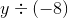(B)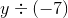(A) and (B) are equal

(B) is greater

(A) is greater

It is impossible to determine which is greater from the information given

(B) is greater

Explanation:

The quotient of two negative numbers is positive. The expressions can be rewritten as follows: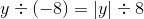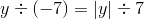Both expressions have the same dividend; the second has the lesser divisor so it has the greater quotient. This makes (B) greater.

### Example Question #23 : Operations

When evaluating the expression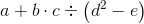,

assuming you know the values of all five variables, what is the second operation that must be performed?

Multiplication

Squaring

Subtraction

Division

Subtraction

Explanation:

In the order of operations, any expression within parentheses must be performed first. Between the parentheses, there are two operations, an exponentiation (squaring), and a subtraction. By the order of operations, the exponentiation is performed first; the subtraction is performed second, making this the correct response.

### Example Question #24 : Operationsis a negative number.

Which is the greater quantity?

(a) The reciprocal of(b) The reciprocal of(b) is the greater quantity

(a) is the greater quantity

It is impossible to determine which is greater from the information given

(a) and (b) are equal

(b) is the greater quantity

Explanation:

Sinceis negative, its reciprocal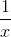is also negative. Since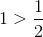,

by the Multiplication Property of Inequality,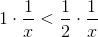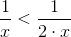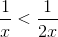That is, the reciprocal ofis greater than that of.

### Example Question #8 : How To Divide Variables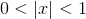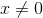.

Which is the greater quantity?

(a) The reciprocal of(b) The reciprocal of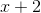(b) is the greater quantity

(a) and (b) are equal

(a) is the greater quantity

It is impossible to determine which is greater from the information given

It is impossible to determine which is greater from the information given

Explanation:

We show that the given information is insufficient by examining two cases.

Case 1: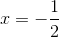The reciprocal ofis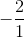, or.

Also,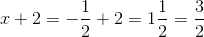, the reciprocal of which is.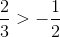, so (b) is the greater quantity.

Case 2: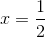.

The reciprocal ofis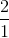, or 2.

Also,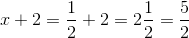, the reciprocal of which is.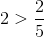, so (a) is the greater quantity.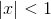in both cases, but in one case, (a) is greater and in the other, (b) is greater.

### Example Question #25 : Variables

Simplify: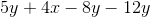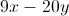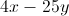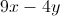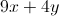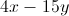Explanation: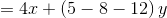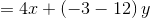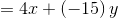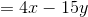### All ISEE Upper Level Quantitative Resources